# A Solution for Binding Silverlight DataGrid Columns to 2nd Level Collection

Supreet Tare posted a thread on MSDN Forum to help him binding a 2nd Level Collection in Silverlight DataGrid control. As he didn't find any good solution there, he asked me on Twitter to give a solution to him for the same. I did a small investigation and came up with a solution for him.

In this post, I am going to describe the problem, as well as solution to this. Hope this solution will be helpful to you too and hence sharing in my blog. Read more to find out the solution.

## Problem Statement

Suppose we have a collection of Students which includes a string property called "Firstname" and another property called "Marks" of type Collection. Each Mark consists of Subject and Subject Mark for example. See the collection structure as shown below: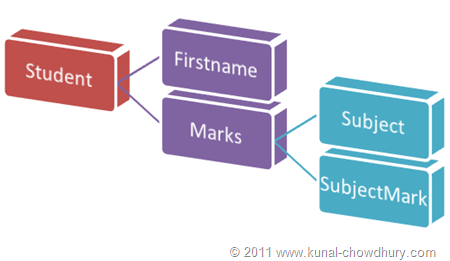Now, if we assign the Student collection to a DataGrid directly, it shows the data as below: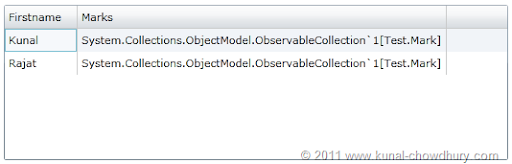So, we need a solution that will show each of the 2nd level items as a separate single column like this: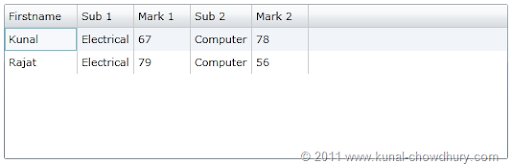## Solution

Here is my solution for the problem statement. I created an ObservableCollection<Student> with the following structure: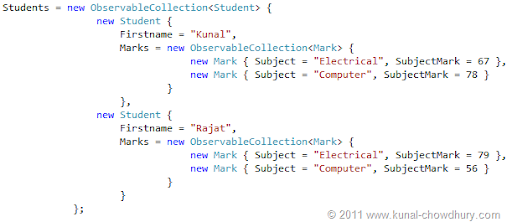We need Value Converters now which will return proper Subject and Mark extracting from the Marks collection.

To do this, we need to create two converters. The first one will take the Marks collection as value, index no. as parameter and return the proper subject name. Here is the code implementation of the same: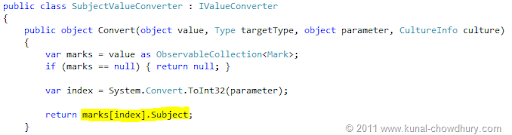Similarly, we need another converter to return the Mark from the collection based on the index no. Let's see the implementation of the converter here: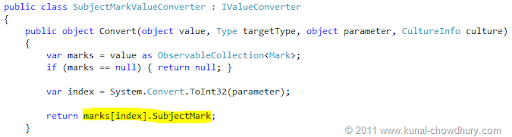Once done, we need to use those converters in our XAML page for DataGrid column. First we will add the those converters as the static resource to the page. We will add the Students collection as the ItemsSource to the DataGrid and set the AutoGenerateColumns property of the DataGrid to false. As this will not create the columns automatically, we need to add the columns manually.

Let's add the columns one by one. The first column is the "Firstname" which is very easy to integrate. For the rest of the columns we will do a small trick to bind the 2nd level collection. Have a look into the following code:

` `
`<UserControl.Resources>`
`    <Test:SubjectValueConverter x:Key="SubjectValueConverter"/>`
`    <Test:SubjectMarkValueConverter x:Key="SubjectMarkValueConverter"/>`
`</UserControl.Resources>`
` `
`<Grid x:Name="LayoutRoot" Background="White">`
`    <sdk:DataGrid Margin="8,46,8,53" ItemsSource="{Binding Students, ElementName=userControl}"`
`                                     AutoGenerateColumns="False">`
`        <sdk:DataGrid.Columns>`
`            <sdk:DataGridTextColumn Header="Firstname"`
`                Binding="{Binding Firstname}"/>`
`            <sdk:DataGridTextColumn Header="Sub 1" `
`                Binding="{Binding Marks, Converter={StaticResource SubjectValueConverter}, ConverterParameter=0}"/>`
`            <sdk:DataGridTextColumn Header="Mark 1" `
`                Binding="{Binding Marks, Converter={StaticResource SubjectMarkValueConverter}, ConverterParameter=0}"/>`
`            <sdk:DataGridTextColumn Header="Sub 2"`
`                Binding="{Binding Marks, Converter={StaticResource SubjectValueConverter}, ConverterParameter=1}"/>`
`            <sdk:DataGridTextColumn Header="Mark 2"`
`                Binding="{Binding Marks, Converter={StaticResource SubjectMarkValueConverter}, ConverterParameter=1}"/>`
`        </sdk:DataGrid.Columns>`
`    </sdk:DataGrid>`
`</Grid>`

From the above code you can see that, we did data binding to Marks collection for rest of the columns. We added the proper converters to the binding with respect to the return type i.e. Subject and Subject Mark. We added a converter parameter which sets the index of Mark in the said collection. For subject 1 and mark 1, we will pass '0' as the converter parameter. For subject 2 and mark 2, we will pass '1' as the converter parameter and so on...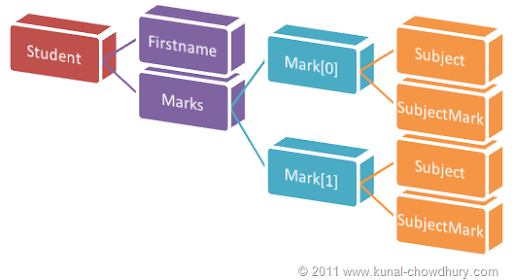Now if you run the application, you will see proper value as shown below:Hope this will help you if you are facing the similar issue. Let me know, if you have further queries on the same. Also, if you have any other solution to that, please let me know.Latest Tech News